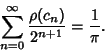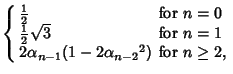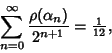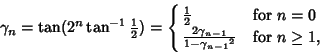## Plouffe's Constant

N.B. A detailed on-line essay by S. Finch was the starting point for this entry.

Define the function(1)

Let(2)

then(3)

For(4)

and(5)

Letting(6)

then(7)

Plouffe asked if the above processes could be inverted.'' He considered(8)

giving(9)

and(10)

giving(11)

and(12)

giving(13)

The latter is known as Plouffe's constant (Plouffe 1997). The positions of the 1s in the Binary expansion of this constant are 3, 6, 8, 9, 10, 13, 21, 23, ... (Sloane's A004715).

Borwein and Girgensohn (1995) extended Plouffe'sto arbitrary Real, showing that if(14)

then(15)

Borwein and Girgensohn (1995) also give much more general recurrences and formulas.

References

Borwein, J. M. and Girgensohn, R. Addition Theorems and Binary Expansions.'' Canad. J. Math. 47, 262-273, 1995.

Finch, S. Favorite Mathematical Constants.'' http://www.mathsoft.com/asolve/constant/plff/plff.html

Plouffe, S.. The Computation of Certain Numbers Using a Ruler and Compass.'' Dec. 12, 1997. http://www.research.att.com/~njas/sequences/JIS/compass.html.

Sloane, N. J. A. Sequence A004715 in The On-Line Version of the Encyclopedia of Integer Sequences.'' http://www.research.att.com/~njas/sequences/eisonline.html.